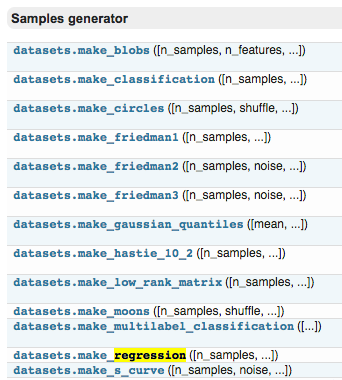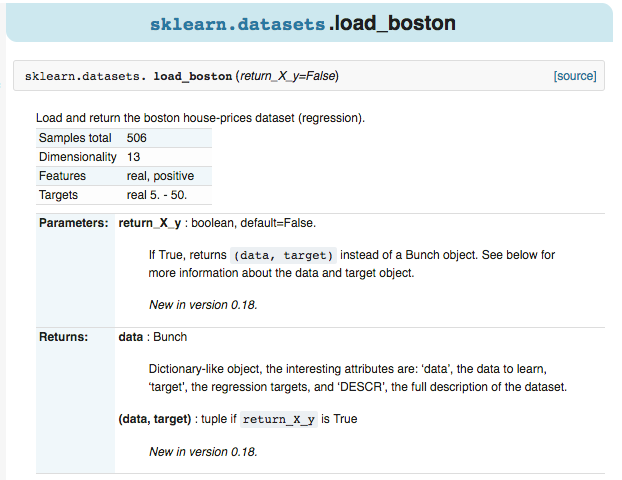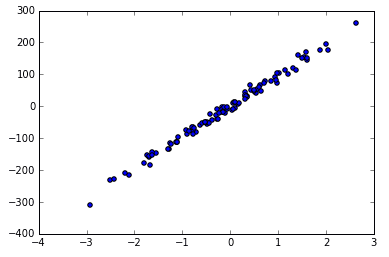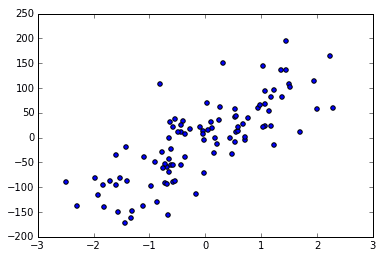# sklearn 强大数据库

## 要点 ¶

eg: boston 房价, 糖尿病, 数字, Iris 花。sklearn.datasets.make_regression(n_samples=100, n_features=100, n_informative=10, n_targets=1, bias=0.0, effective_rank=None, tail_strength=0.5, noise=0.0, shuffle=True, coef=False, random_state=None)[source]


## 导入模块 ¶

from __future__ import print_function
from sklearn import datasets
from sklearn.linear_model import LinearRegression
import matplotlib.pyplot as plt


## 导入数据－训练模型 ¶

datasets.load_boston() 的形式加载数据，并给 Xy 赋值，这种形式在 Sklearn 中都是高度统一的。

loaded_data = datasets.load_boston()


model = LinearRegression()
model.fit(data_X, data_y)


print(model.predict(data_X[:4, :]))
print(data_y[:4])

“”“
[ 30.00821269  25.0298606   30.5702317   28.60814055]
[ 24.   21.6  34.7  33.4]
”“”


## 创建虚拟数据－可视化 ¶

X, y = datasets.make_regression(n_samples=100, n_features=1, n_targets=1, noise=10)


scatter 的形式来输出结果。

plt.scatter(X, y)
plt.show()noise 越大的话，点就会越来越离散，例如 noise 由 10 变为 50.

X, y = datasets.make_regression(n_samples=100, n_features=1, n_targets=1, noise=50)
plt.scatter(X, y)
plt.show()